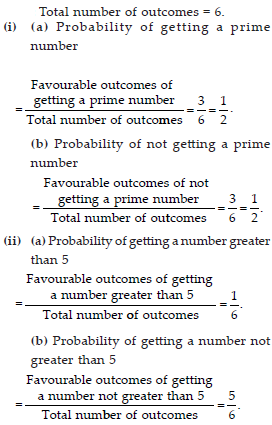# Data Handling (Mathematics) Class 8 - NCERT Questions

Q 1.

For which of these would you use a histogram to show the data?
(A) The number of letters for different area in postman’s bag.
(B) The height of competitors in an athletics meet.
(C) The number of cassettes produced by 5 companies.
(D) The number of passengers boarding trains from 7:00 a.m. to 7:00 p.m. at a station.
Give reasons for each.

SOLUTION:

(a) We use pictograph for the given statement. Since, letters can be represented through appropriate picture or symbols, we will not use histogram.
(b) We use histogram for the given statement since the height of competitors in an athletics meet can be divided into class intervals.
(c) We will not use histogram. We use pictograph for the given statement since the cassettes can be represented through appropriate picture or symbols.
(d) We use histogram for the given statement since the time of boarding trains from 7:00 a.m. to 7:00 p.m. can be divided into class intervals.

Q 2.

The shoppers who come to a departmental store are marked as : man (M), woman (W), boy (B) or girl (G). The following list gives the shoppers who came during the first hour in the morning :
W W W G B W W M G G M M W W W W G B M W B G G M W W M M W W W M W B W G M W W W W G W M M W W M W G W M G W M M B G G W
Make a frequency distribution table using tally marks. Draw a bar graph to illustrate it.

SOLUTION:

Firstly by using a given information, we make a frequency distribution, table by using tally marks.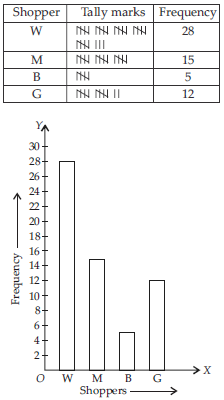Q 3.

The weekly wages (in Rs.) of workers in a factory are:
830, 835, 890, 810, 835, 836, 869, 845, 898, 890, 820, 860, 832, 833, 855, 845, 804, 808, 812, 840, 885, 835, 835, 836, 878, 840, 868, 890, 806, 840.
Using tally marks make a frequency table with intervals as 800 – 810, 810 – 820 and so on.

SOLUTION:

By using a given information we have to make a frequency table :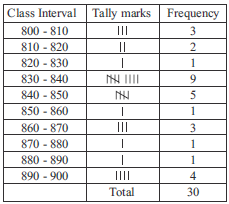Q 4.

Draw a histogram for the frequency table made for the data in Question 3, and answer the following questions.
(i) Which group has the maximum number of workers?
(ii) How many workers earn Rs. 850 and more?
(iii) How many workers earn less than Rs. 850?

SOLUTION: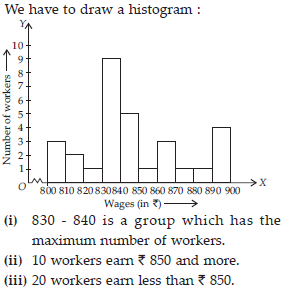Q 5.

The number of hours for which students of a particular class watched television during holidays is shown through the given graph. Answer the following :
(i) For how many hours did the maximum number of students watch TV?
(ii) How many students watches TV for less than 4 hours?
(iii) How many students spent more than 5 hours in watching TV?

SOLUTION:

(i) The above given graph shows that in between 4 - 5 hours, maximum number of students used to watch T.V.
(ii) Number of students watched T.V. for less than 4 hours = 4 + 8 + 22 = 34.
(iii) Number of students spent more than 5 hours in watching T.V. = 8 + 6 = 14.

Q 6.

A survey was made to find the type of music that a certain group of young people liked in a city. Adjoining pie chart shows the finding of this survey.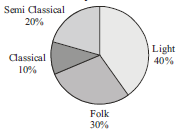From this pie chart answer the following :
(i) If 20 people liked classical music, how many young people were surveyed?
(ii) Which type of music is liked by the maximum number of people?
(iii) If a cassette company were to make 1000 CD’s, how many of each type would they make?

SOLUTION: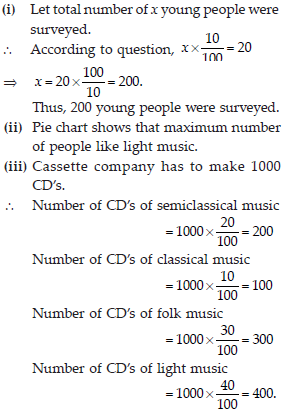Q 7.

A group of 360 people were asked to vote for their favourite season from the three seasons rainy, winter and summer.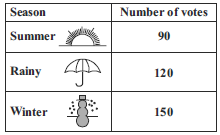(i) Which season got the most votes?
(ii) Find the central angle of each sector.
(iii) Draw a pie chart to show this information.

SOLUTION: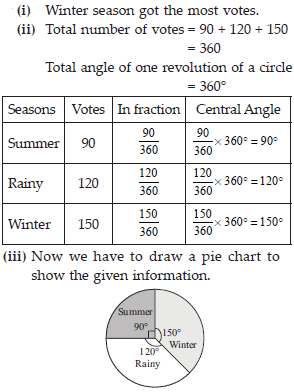Q 8.

Draw a pie chart showing the following information. The table shows the colours preferred by a group of people.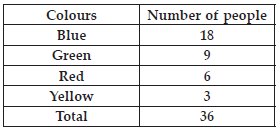Find the proportion of each sector. For example, Blue is 18/36 = 1/2; Green 9/36 = 1/4 and so on. Use this to find the corresponding angles.

SOLUTION: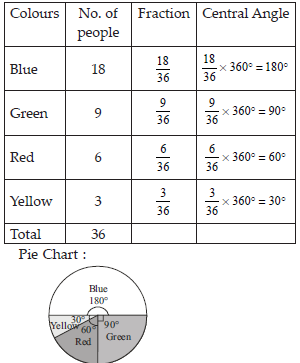Q 9.

The given pie chart gives the marks scored in an examination by a student in Hindi,English, Mathematics, Social Science and Science. If the total marks obtained by the students were 540, answer the following questions.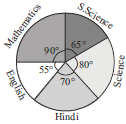(i) In which subject did the student score 105 marks?
(ii) How many more marks were obtained by the student in Mathematics than in Hindi?
(iii) Examine whether the sum of the marks obtained in Social Science and Mathematics is more than that in science and Hindi.

SOLUTION: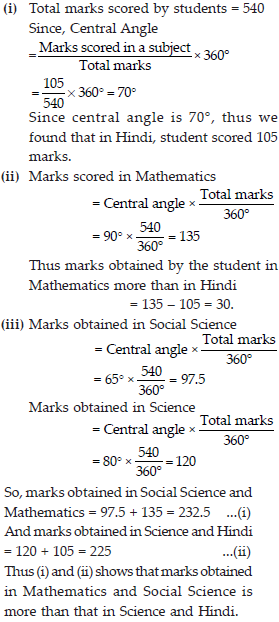Q 10.

The number of students in a hostel, speaking different languages is given below. Display the data in a pie chart.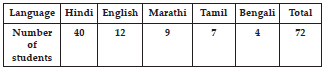SOLUTION: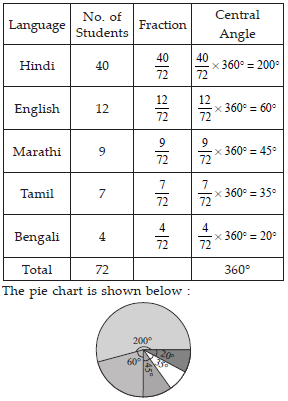Q 11.

List the outcomes you can ABCDA see in these experiments.
(A) Spinning a wheel
(B) Tossing two coins together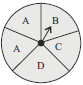SOLUTION:

(a) After spinning a wheel, we obtained total outcomes: A, B, C and D.
(b) After tossing two coins together, we get the following outcomes:
(i) HT. i.e., Head on first coin and Tail on the second coin.
(ii) HH. i.e., Head on both the coins.
(iii) TH. i.e., Tail on first coin and Head on second coin.
(iv) TT. i.e., Tail on both the coins.

Q 12.

When a die is thrown, list the outcomes of an event of getting
(i) (A) a prime number
(B) not a prime number
(ii) (A) a number greater than 5
(B) a number not greater than 5.

SOLUTION:

Since total numbers on a die are 1, 2, 3, 4, 5 and 6.
After throwing a die, we get
(i) (a) 2, 3, 5 are prime numbers.
(b) 1, 4, 6 are not prime numbers.
(ii) (a) 6 is a number greater than 5.
(b) 1, 2, 3, 4, 5 are not greater than 5.

Q 13.

Find the
(A) Probability of the pointer stopping on D in (Question 1 (A)?
(B) Probability of getting an ace from a well shuffled deck of 52 playing cards?
(C) Probability of getting a red apple. (See figure below).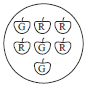SOLUTION: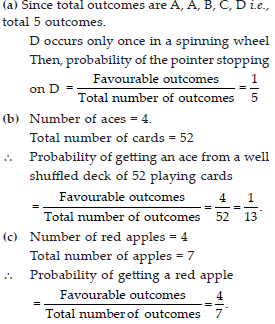Q 14.

Numbers 1 to 10 are written on ten separate slips (one number on one slip), kept in a box and mixed well. One slip is chosen from the box without looking into it. What is the probability of
(i) getting a number 6?
(ii) getting a number less than 6?
(iii) getting a number greater than 6?
(iv) getting a 1-digit number?

SOLUTION: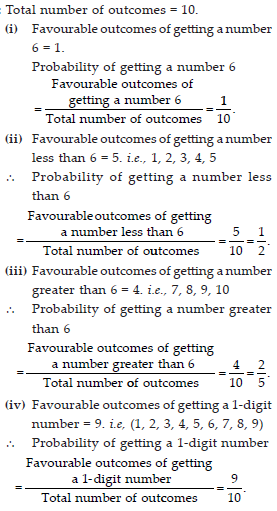Q 15.

If you have a spinning wheel with 3 green sectors, 1 blue sector and 1 red sector, what is the probability of getting a green sector? What is the probability of getting a non blue sector?

SOLUTION: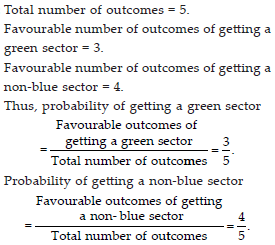Q 16.

Find the probability of the events given in Question 2.

SOLUTION: